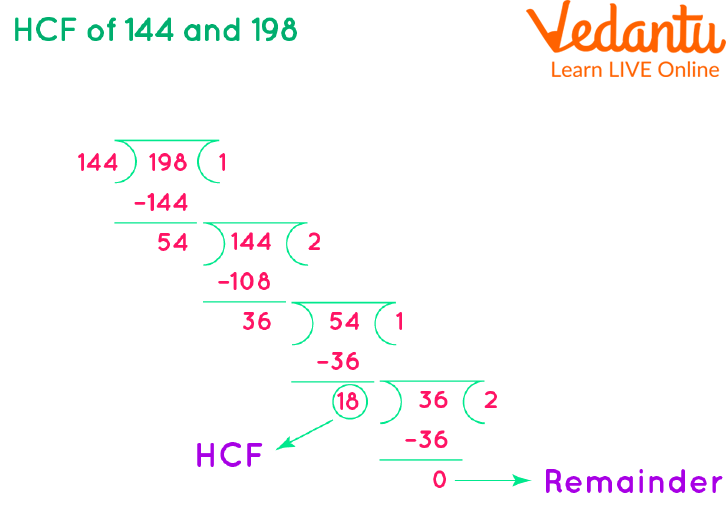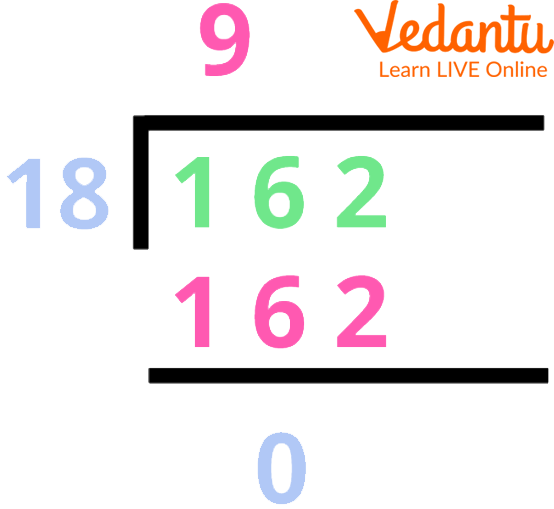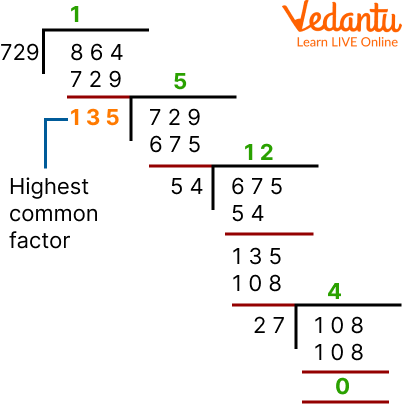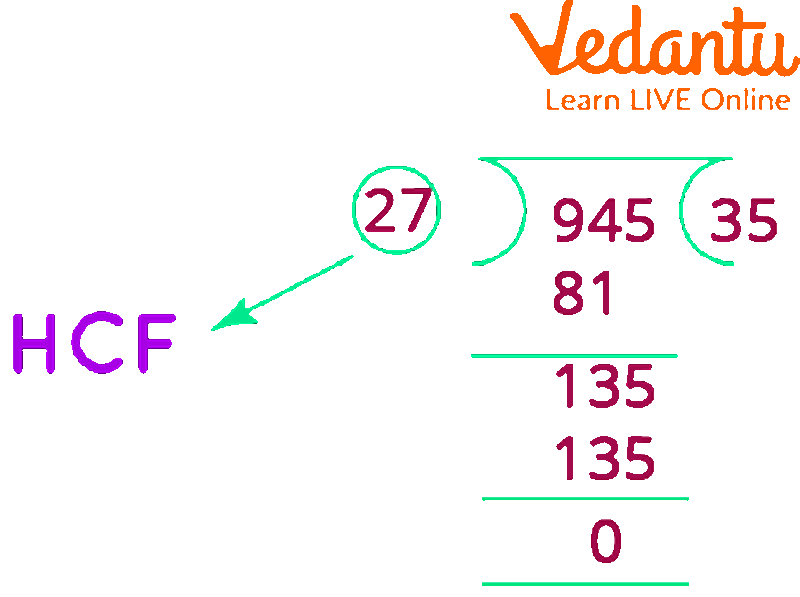Courses
Courses for Kids
Free study material
Free LIVE classes
More

# How to Find HCF of 3 Numbers?LIVE
Join Vedantu’s FREE Mastercalss

## Introduction to HCF

The highest number that completely divides two numbers is known as the Highest Common Factor (HCF). The Greatest Common Divisor (GCD) is another name for the Highest Common Factor (HCF) (GCD). The HCF of two numbers can be determined in a variety of methods. Using the prime factorization method is one of the easiest ways to get the HCF of two or more numbers. In this article, we will learn how to find HCF by long division method with some examples.

## Steps to Find HCF of 3 Numbers by Using the Long Division Method

Follow the Steps for a better understanding:

Step 1: We first need to find out the HCF of the first two given numbers.

Step 2: Find out the HCF of the third number given and the HCF of the first two numbers that we got from the previous step.

Step 3: We mark the highest factor among which we found common.

## Solved Examples

Q1. Find the HCF of 144, 198 and 162

Ans: Using the long division method

First of all, arrange the given numbers in ascending order. Not necessary but preferable.

Let's first find out the HCF of the first 2 numbers which are 144 and 198. i.e. HCF of 144 and 198.HCF of 144 and 198 by Using Long Division Method

Therfore, the HCF of 144 and 198 = 18

The next step is to find the HCF of 162 and 18HCF of 162 and 18

So by applying HCF division method, we get HCF of 162 and 18 = 18

Therefore the HCF of all the 3 numbers is

HCF of 144, 198 and 162 = 18

Note: It's Important that the Remainder is Always 0 at the End

Let's try to understand with one more example

Q2. Find the HCF of 729, 864 and 945

Ans: Using the long division method

Let's first find out the HCF of the first 2 numbers which are 729 and 864. i.e. HCF of 864 and 729.HCF of 864 and 729

The HCF of HCF of 864 and 729 = 27

The next step is to find the HCF of the third number that is given which is 945 and the HCF of the first two numbers which is 27.HCF of 945 and 27

HCF of 945 and 27 = 27

Therefore the HCF of all the 3 numbers is 27.

HCF of 729, 864 and 945 = 27

## Practice Problem

Q1. Find the HCF of 30, 45 and 150

Ans: 15

Q2. Find the HCF of 126, 162 and 180

Ans: 18

Q3. Find the HCF of 300, 270 and 9

Ans: 3

Q4. Find the HCF of 78, 98 and 108

Ans: 2

Q5. Find the HCF of 702, 153 and 405

Ans: 9

## Summary

This article has tried to explain the detailed steps of how to find HCF by using the long Division method for 3 given numbers. To find the HCF by division method the first step is to divide the larger number by the smaller number and then the remainder becomes the divisor and divide the smaller number until the remainder is zero. So, read the whole content nicely and do the solved examples present here in this article.

Last updated date: 29th Sep 2023
Total views: 107.7k
Views today: 1.07k

## FAQs on How to Find HCF of 3 Numbers?

1. What is the importance of HCF?

HCF is mainly used in mathematical problems to distribute things into smaller sections. It could be used to arrange something into smaller groups for distribution and can also be applied in our daily lives as well.

2. Can the HCF of any given number be 0 or 1?

It is possible that the HCF of the given figures can be 1 only if the given figures don’t have any other common factors apart from 1.  For example: HCF of (2,3) = 1,  HCF of (3,4) = 1, HCF of (4,5)= 1, HCF of (5,6) = 1, HCF of (9,10) = 1.  It is possible to have the HCF of given figures to have 0 only if all the given figures are 0 because every natural number is a common divisor of 0 and 0, and therefore HCF (0,0) = 0.

3. How is HCF calculated?

There are several methods through which we can calculate HCF which are (1) prime factorization method (2) factorization method (3) short division method.  We can use any one of them whatever we feel is easy to calculate.